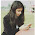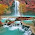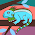## Monday, 27 May 2013

### CBSE Class 10 - Science - CHEMICAL REACTIONS AND EQUATIONS (MCQs)

CHEMICAL REACTIONS AND EQUATIONS

MCQs

Q1: Which of the following is not a decomposition reaction?

(a) CaCO3 → CaO + CO2
(b) H2 + Cl2 → 2HCl
(c) H2CO3 → H2O + CO2
(d) 2KClO3 → 2KCl + 3O2

Q2: Which of the following is not an oxidising agent?

(a) Oxygen
(b) Conc. Sulphuric acid
(c) Chlorine
(d) Hydrogen

Q3: The oxidation reaction which produces heat and light is

(a) endothermic
(b) photochemical
(c) combustion
(d) exothermic

Q4: 2Pb(NO3)2 → 2PbO + nA + O2
What is nA in the given reaction?

(a) 4NO
(b) 4NO2
(c) 2PbNO2
(d) NO2

Q5: A slow combustion in which glucose present in the body cells combine with oxygen to provide energy is

(a) digestion
(b) excretion
(c) respiration
(d) none of the above

Q6: The equation
Cu + XHNO3 → Cu(NO3)2 + YNO2 + 2H2O, the value of X and Y are

(a) 3 and 1 respectively
(b) 8 and 6 respectively
(c) 4 and 2 respectively
(d) 7 and 1 respectively

Q7: When the gases sulphur dioxide and hydrogen sulphide mix in the presence of water, the reaction SO2 + 2H2S → 2H2O + 3S occurs. Here hydrogen sulphide is acting as

(a) an oxidising agent
(b) a reducing agent
(c) a dehydrating agent
(d) a catalyst

Q8: What is the chemical name of quick lime ?

(a) Calcium oxide
(b) Calcium carbonate
(c) Calcium hydroxide
(d) Carbon dioxide

Q9:Which of the following reaction will not take place?

(a) Zn + FeSO4 → ZnSO4 + Fe
(b) 2KI + Cl2 → 2KCl + I2
(c) Zn + MgSO4 → ZnSO4 + Mg
(d) Mg + CuSO4 → MgSO4 + Cu

Q10: In the reaction, 2FeCl2 + Cl2 → 2FeCl3 , chlorine may be regarded as

(a) an oxidizing agent
(b) a reducing agent
(c) a catalyst
(d) providing an inert medium

Q11: The conversion of K2Cr2O7 into Cr2(SO4)3 is a process of

(a) Oxidation
(b) Reduction
(c) Decomposition
(d) Substitution

Q12: An element, which never has a positive oxidation state in any of its compounds, is

(a) Boron
(b) Oxygen
(c) Chlorine
(d) Fluorine

Q13: Amino acid is formed by decomposition of which component of our diet?
(a) Carbohydrate
(b) Starch
(c) Protein
(d) Fat

Q14: Loss of electrons is called _________

(a) reduction
(b) oxidation
(c) can be oxidation or reduction
(d) none of these

Answers:
1: (b) H2 + Cl2 → 2HCl
2: (d) Hydrogen
3: (c) combustion
4: (b) 4NO2
5: (c) respiration
6: (c) 4 and 2 respectively
7: (b) a reducing agent
8: (a) Calcium oxide
9: (c) Zn + MgSO4 → ZnSO4 + Mg
10: (a) an oxidizing agent
11: (b) Reduction
12: (d) Fluorine
13: (c) Protein
14: (b) oxidation#### 9 comments:

1.Helped me a lot :)

2.good going this site is very very......good

3.4 question is wrong its option should be 4NO2

1.Thanks Typo corrected.

4.plz tell me in que.10 why chrorine is oxidising agent bcoz there r no molecules of O2

5.plz tell me in que.10 why chrorine is oxidising agent bcoz there r no molecules of O2

1.Loss of electronegative radical or gain of +ve radical is also reduction. Thus cl2 acts as oxidising agent.

6.Want more MCQs

7.Want more MCQs

We love to hear your thoughts about this post!